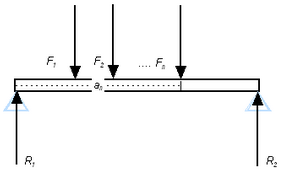## Beam Supports calculatorLength of beam (m, ft):

Dist. from R1 (m, ft) Force F1 (N, lbf):
Dist. from R1 (m, ft) Force F2 (N, lbf):
Dist. from R1 (m, ft) Force F3 (N, lbf):
Dist. from R1 (m, ft) Force F4 (N, lbf):
Dist. from R1 (m, ft) Force F5 (N, lbf):
Dist. from R1 (m, ft) Force F6 (N, lbf):

Beam Supports formula:

F1 af1 + F2 af2 + .... + Fn afn = R ar1 + R ar2

where R = force from support (N, lbf), F = force from load (N, lbf), a = the distance from the force to a common reference.

Thinkcalculator.com provides you helpful and handy calculator resources.